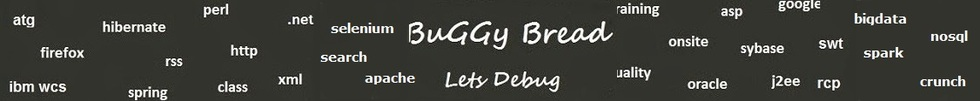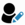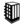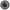Write a program for binary tree ?# Search Interview QuestionsMore than 3000 questions in repository.There are more than 900 unanswered questions.Have a video suggestion.
Click Correct / Improve and please let us know.
Label / Company      Label / Company / TextSubmit Question

Java - Interview Questions and AnswersQ1. Write a program for binary tree ?Data Structure2017-09-15 08:00:14Ans. public class BinarySearchTree {
//Represent the node of binary tree
public static class Node {
int data;
Node left;
Node right;
public Node(int data) {
//Assign data to the new node, set left and right children to null

this.data = data;
this.left = null;
this.right = null;
}
}
//Represent the root of binary tree

public Node root;
public BinarySearchTree() {
root = null;
}

//factorial() will calculate the factorial of given number

public int factorial(int num) {
int fact = 1;
if (num == 0) return 1;
else {
while (num > 1) {
fact = fact * num;
num--;
}
return fact;
}
}

//numOfBST() will calculate the total number of possible BST by calculating Catalan Number for given key

public int numOfBST(int key) {
int catalanNumber = factorial(2 * key) / (factorial(key 1) * factorial(key));
return catalanNumber;
}
public static void main(String[] args) {
BinarySearchTree bt = new BinarySearchTree();

//Display total number of possible binary search tree with key 5

System.out.println("Total number of possible Binary Search Trees with given key: "
bt.numOfBST(5));
}
}Help us improve. Please let us know the company, where you were asked this question :LikeDiscussCorrect / ImproveAsked in 1 CompaniesRelated QuestionsWhat is a binary tree ?Write code for Binary tree traversal ?Flatten a Binary Tree to Linked ListWrite code to check if a Binary tree is symmetricalWrite program to balance a binary treeGiven a sorted array, write an algorithm to create a binary tree.Write a Program to find depth of node binary tree ?

## Help us and Others Improve. Please let us know the questions asked in any of your previous interview.

Any input from you will be highly appreciated and It will unlock the application for 10 more requests.